# How to Extract Data Which is Greater Than Value1 but Less Than Value2 in Excel

Sometimes in a column lists some numbers we need to filter values which are greater than one certain value and at the same time less than another value, and then extract them into another column. In this situation we can use Filter function to create number filter to filter data properly. In this article, we will show you the details to create number filter step by step.

Precondition:

See table below. If we want to extract ID which score is in level B (greater than 69 and less than 85), we need to create number filter here.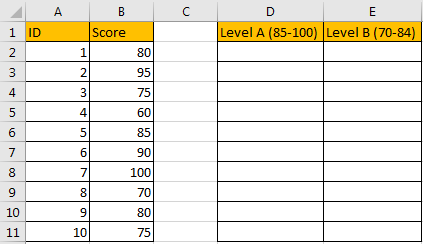## Method: Extract Data by Create Number Filter in Excel

Step 1: Create a filter on column B. Select B1, click Data in ribbon, click Filter.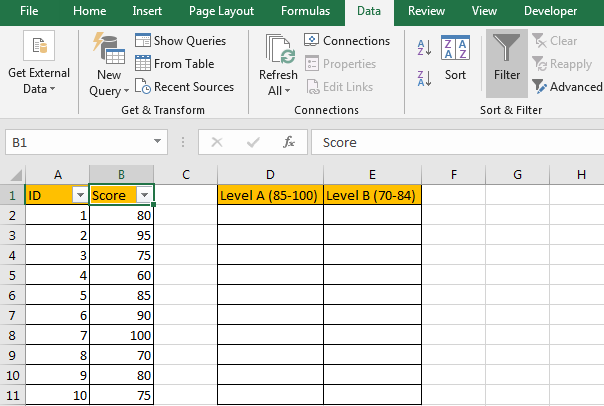Step 2: On B1 click Arrow button, then in filter menu click on Number Filters->Customer Filter.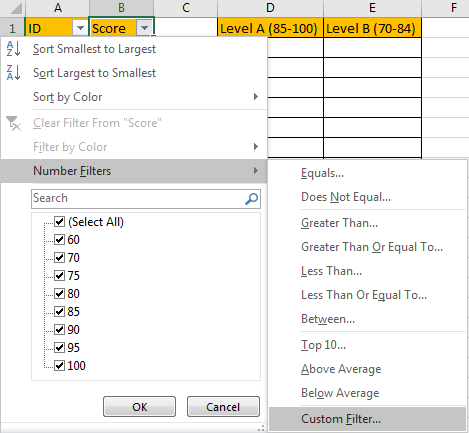Step 3: In Customer AutoFilter dialog, select ‘is greater than’ in dropdown list 1, then enter 69 as criteria in adjacent dropdown list; check on ‘And’, select ‘is less than’ in dropdown list 2, and then enter 85 as criteria in adjacent dropdown list.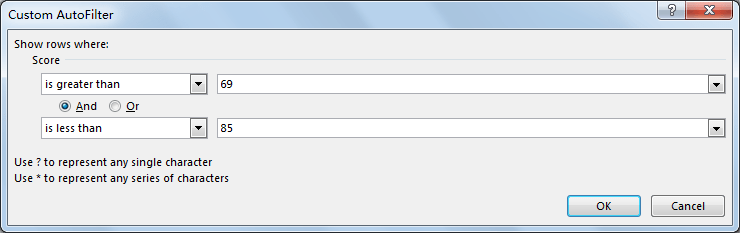Step 4: Click OK. Verify that matched criteria values are filtered.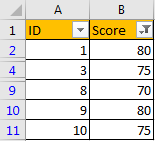Step 5: Press F5 to trigger Go To dialog.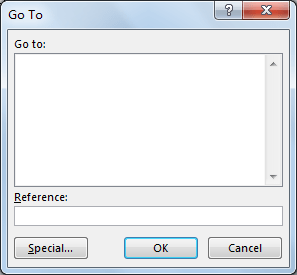Step 6: Click Special to load ‘Go To Special’ dialog, check on ‘Visible cells only’. Click OK.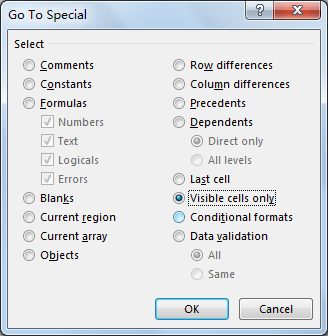Step 7: On column A, select the visible range, press Ctrl+C to copy visible cells, then paste them in another worksheet.

Step 8: Clear filter, all rows are expanded properly. Then copy values from another worksheet back to Level B column properly. Now all IDs match level B criteria are extracted.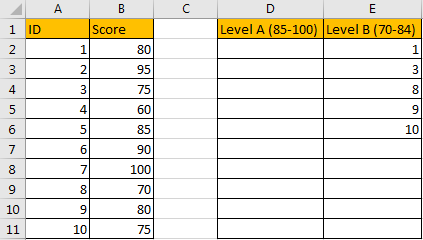Related Posts

If Cell is This Value or That Value

IF function is frequently used in Excel worksheet to return you expect “true value” or “false value” based on the result of logical test. If you want to see if a cell is A or B, and if one of ...

If Value is Greater Than A Certain Value

IF function is frequently used in Excel worksheet to return you expect “true value” or “false value” based on the logical test result. If you want to see if a value in one cell is greater than a specific value, ...

If Cell is Not Blank

IF function is frequently used in Excel worksheet to return you expect “true value” or “false value” based on the result of created logical test. If you want to see if a cell is blank or not, and leave some ...

VBA Macro For VLOOKUP From Another Sheet

In the previous post, you should know that how to fix or remove the #N/A error when using VLOOKUP formula to lookup value from another sheet. And this post will show you how to use VBA code to vlookup data ...

If Cell is Blank

IF function is frequently used in Excel worksheet to return you expect “true value” or “false value” based on the result of created logical test. If you want to see if a cell is blank or not, and leave some ...

If Cell Equals Certain Text String

IF function is frequently used in Excel worksheet to return you expect “true value” or “false value” based on the result of created logical test. If you want to see if cell equals a certain text string like “Win”, you ...

If Cell Contains Either Text1 or Text2

IF function is frequently used in Excel worksheet to return “true value” or “false value” based on the logical test result. If you want to see if cell contains certain substring1 like “abc” or substring2 like “def”, and returns true ...

If Cell Contains Certain Text OR Equals Certain Text

IF cell equals certain text IF function is frequently used in Excel worksheet to return “true value” or “false value” based on the logical test result. If you want to test values to see if they equal certain text like ...

VLOOKUP From Another Sheet Not Working

In the previous post, you should know that how to fix or remove the #N/A error when using VLOOKUP formula to lookup value from another sheet. And this post will show you reasons why your VLOOKUP formula is not working ...

If Cell Begins with One of Three Supplied Characters

If you want to test values to see if they begin with some given specific characters like “x”, ”y”, or “z”, you can create a formula with COUNTIF and SUM functions to return results. EXAMPLE You can see “TRUE” or ...

Sidebar